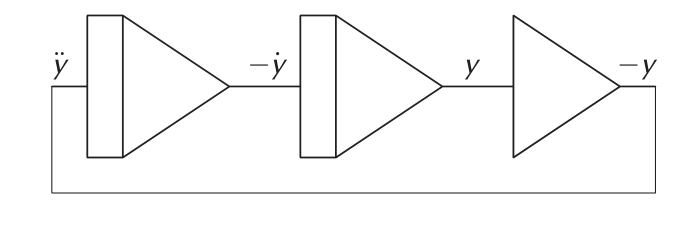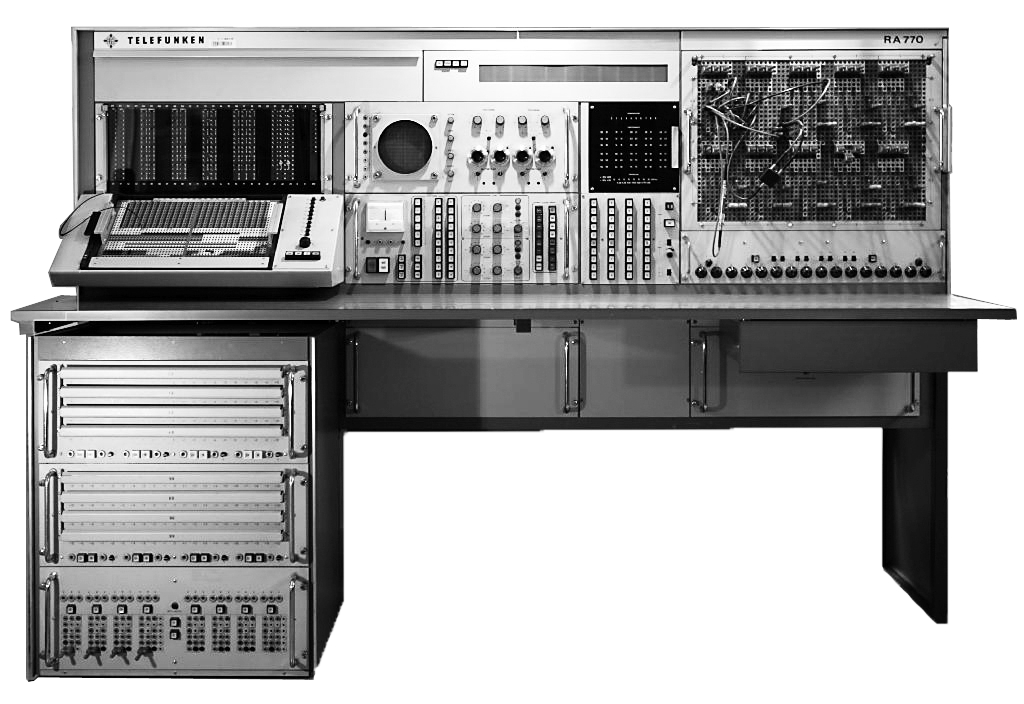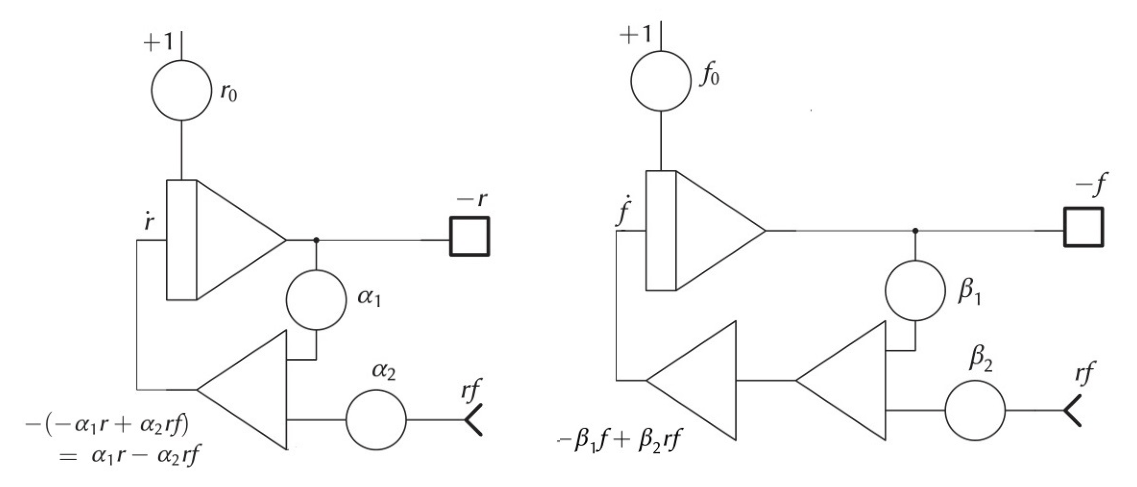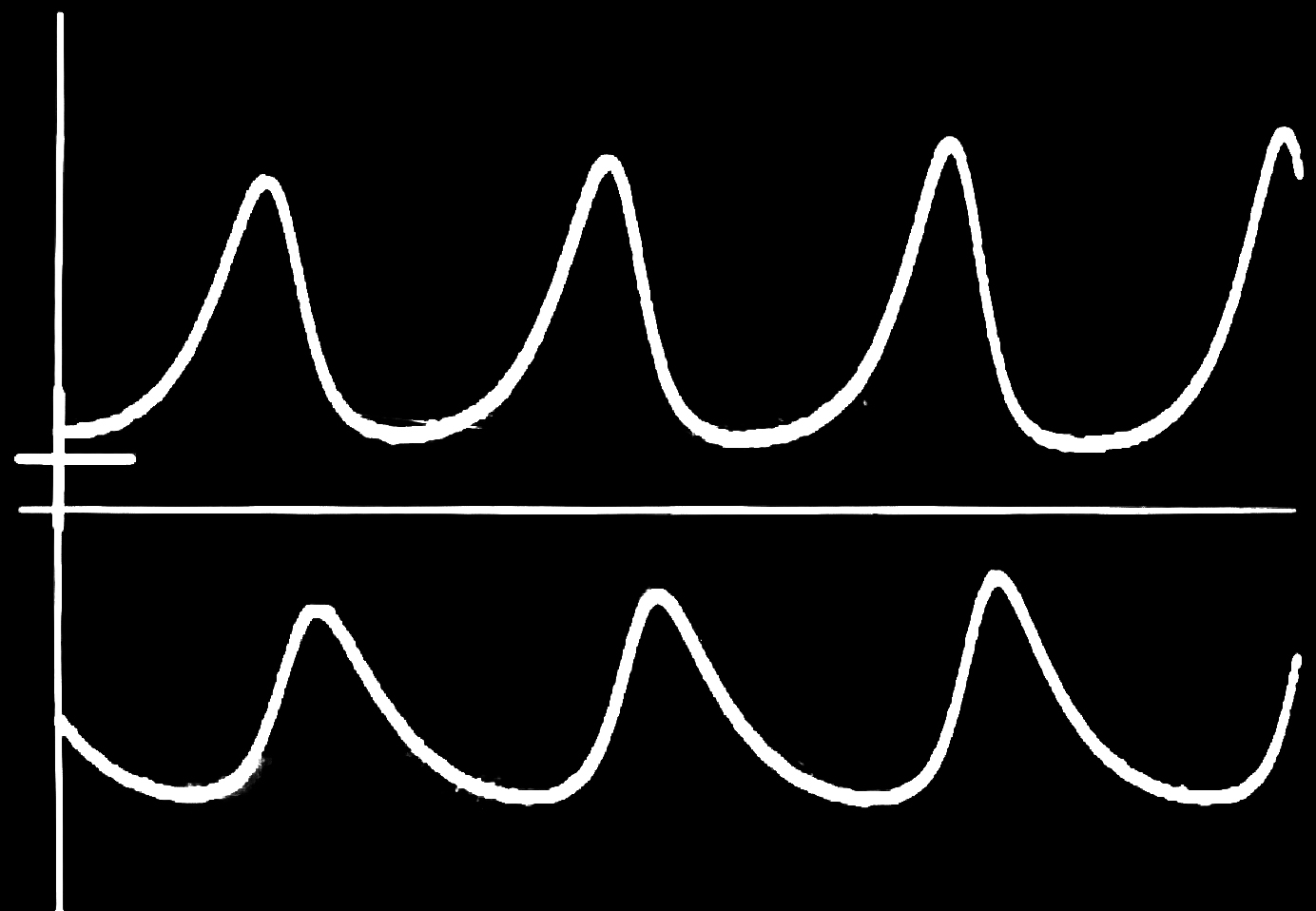# Analogue computing: fun with differential equations

Solving differential equations instantaneously, using some electrical components and an oscilloscopeWhen it comes to differential equations, things start to get pretty complicated—or at least that’s what it looks like. When I studied mathematics, lectures on differential equations were considered to be amongst the hardest and most abstract of all and, to be honest, I feared them because they really were incredibly formalistic and dry. This is a pity as differential equations make nature tick and there are few things more fascinating than them.

When asked about solving differential equations, most people tend to think of a plethora of complex numerical techniques, such as Euler’s algorithm, Runge–Kutta or Heun’s method, but few people think of using physical phenomena to tackle them, representing the equation to be solved by interconnecting various mechanical or electrical components in the right way. Before the arrival of high-performance stored-program digital computers, however, this was the main means of solving highly complicated problems and spawned the development of analogue computers.

## Analogies and analogue computers

When faced with a problem to solve, there are two approaches we could take. The first is to recreate a scaled model of the problem to be investigated, based on exactly the same physical principles as the full size version. This is often done in, for example, structural analysis: Antonì Gaudi first used strings and weights to build a smaller model of his Church of Colònia Güell near Barcelona to help him determine whether it was stable. Similar techniques have been used from the Gothic period well into the 20th century, when a textile fabric was used to design the roof structure for the Olympic stadium in Munich.Gaudi’s structural analysis model of the Colonia Guell.

As powerful as this approach is (as another example, think of using soap films when determining a minimal surface), it is quite limited in its application as you are restricted to the same physical principles as those in the full problem. This is where the second technique comes into play: comparing the potentially very complex system under study to a different, but behaviourally similar, physical system. In other words, this similar, probably simpler, physical system is an analogy of the first: hence the creation and naming of analogue computers—computers that are able to study one phenomenon by using another, such as looking at the behaviour of a mechanical oscillator by using an electronic model.

## Analogue computers

Analogue computers are powerful computing devices consisting of a collection of computing elements, each of which has some inputs and outputs and performs a specific operation such as addition, integration (a basic operation on such a machine!) or multiplication. These elements can then be interconnected freely to form a model, an analogue, of the problem that is to be solved.

The various computing elements can be based on a variety of different physical principles: in the past there have been mechanical, hydraulic, pneumatic, optical, and electronic analogue computers. Leaving aside the Antikythera mechanism—which is the earliest known example of a working analogue computer, used by the ancient Greeks to predict astronomical positions and eclipses—the idea of general purpose analogue computers was developed by William Thomson, better known as Lord Kelvin, when his brother, James Thomson, developed a mechanical integrator mechanism (previously also developed by Johann Martin Hermann in 1814).

Lord Kelvin realised that, given some abstract computing elements, it is possible to solve differential equations using machines: a truly trailblazing achievement. Let us try to solve the differential equation representing simple harmonic motion (perhaps of a mechanical oscillator!),
\begin{align}
\frac{\mathrm{d}^2y}{\mathrm{d}t^2}+\omega^2y=0,\tag{1}
\end{align}
by means of a clever setup consisting of integrators and other devices and using the technique developed by Lord Kelvin in 1876.

We can write (1) more compactly as $\ddot{y}+\omega^2y=0$, where the dots over the variables denote time derivatives. To simplify things a bit we will also assume that $\omega^2=1$. Hence we rearrange (1) so that the highest derivative is isolated on one side of the equation, yielding
\begin{equation}
\ddot{\hspace{-2pt}y}=-y.\tag{2}
\end{equation}

Let us now assume that we already know what $\ddot{y}$ is (a blatant lie, at least for the moment). If we have some device capable of integration it would be easy to generate $\dot{y}=\int\ddot{y}\ \text{d}t+c_0$ and from that $y=\int\dot{y}\ \text{d}t+c_1$, with some constants $c_0$ and $c_1$.

Using a second type of computing element that allows us to change signs, it is therefore possible to derive $-y$ from $\ddot{y}$ by means of three interconnected computing elements (two integrators and a sign changer). Obviously, this is just the right hand side of (2), which is equal to $\ddot{y}$, assumed known at the beginning. Now Kelvin’s genius came to the fore: we can set up a feedback circuit by feeding the first integrator in our setup with the output of the sign changing unit at the end. This is shown below in an abstract (standard) notation: this is how programs for analogue computers are written down.The basic circuit for solving $\ddot{y}=-y$. From left to right we have two integrators and a summer (with each component inverting the sign).

The two triangular elements with the rectangles on their left denote integrators; while the single triangle on the right is a summer. It should be noted that for technical reasons all of these computing elements perform an implicit change of sign, so the leftmost integrator actually yields $-\dot{y}$ instead of $\dot{y}$ as in our thought experiment above, while the summer with the one input $y$ yields $-y$.

However, if one sets up the two integrators and a summer as demonstrated above, the system would just sit there and do nothing, yielding the constant zero function as a solution of the differential equation (2): not an incorrect solution, but utterly boring.

This is where $c_0$ and $c_1$ come into play: these are the initial conditions for the integrators. Let us assume that $c_0=1$ and $c_1=0$, ie the leftmost integrator starts with the value $1$ at its output, which feeds into the second integrator, which in turn feeds the sign changing summer, which then feeds the first integrator. This will result in a cosine signal at the output of the first integrator and a minus sine function at the output of the second one, perfectly matching the analytic solution of (2). Such initial conditions are normally shown as being fed into the top of the rectangular part of an integrator symbol, but we have omitted this in our diagrams.Setup for the predator-prey simulation.

So if we have some computing elements, we have seen that we can arrange them to create an abstract model of a differential equation, giving us some form of specialised computer: an analogue computer! The implementation of these computing elements could be done in different ways: time integration, for example, could be done by using the integrand to control the flow of water into a bottle, or to charge a capacitor, or we could build some other intricate mechanical system. Some of the most important observations to make are the following:

1. Analogue computers are programmed not in an algorithmic fashion but by actually interconnecting their individual computing elements in a suitable way. Thus they do not need any program memory; in fact, there is no “memory” in the traditional sense at all.
2. What makes an analogue computer “analogue” is the fact that it is set up to be an analogy of some problem readily described by differential equations or systems of them. Even digital circuits qualify as analogue computers and are known as Digital Differential Analysers (DDA).
3.  Programming an analogue computer is quite simple (although there are some pitfalls that are beyond the scope of this article). One just pretends that the highest derivative in an equation is known and generates all the other terms from this highest derivative by applying integration, summation, multiplication, etc until the right-hand side of the equation being studied is obtained, with the result then fed into the first integrator.

As a remark it should be noted that Kelvin’s feedback technique, as it is known, can also be applied to traditional stored-program digital computers.

## Examples of analogue computers

Analogue computers were the workhorses of computing from the 1940s to the mid-1980s when they were finally superseded by cheap and (somewhat) powerful stored-program digital computers. Thus without them, the incredible advances in aviation, space flight, engineering and industrial processes after the Second World War would have been impossible. A typical analogue computer of the 1960s was the Telefunken RA 770, shown below.The Telefunken RA 770 analogue computer.

The most prominent feature of such a machine is the patch field, which is on the far right of the picture above. Here all of the inputs and outputs of the literally hundreds of individual computing elements are brought together. Using (shielded) patch cords, these computing elements are connected to each other, setting up the desired model. In the middle are the manual controls (start/stop a computation, set parameter values, etc) and an oscilloscope to display the results as curves. On the upper far left is a digital extension that allows us to set up things like iterative operations, where one part of the computer generates initial conditions for another part. Below left are eight function generators, which can be manually set to generate rather arbitrary functions by a polygonal approximation.

## A more complex example

Let us now look at a somewhat more complex programming example: the investigation of a predator-prey model as described by Alfred James Lotka in 1925 and then Vito Volterra in 1926. This consists of a closed ecosystem with only two species, foxes and rabbits, and an unlimited food supply for the rabbits. Rabbits are removed from the system by being eaten by the foxes—without this mechanism their population would just grow exponentially. Foxes, on the other hand, need rabbits for food, or they would die of starvation. This system can be modelled by two coupled differential equations with $r$ and $f$ denoting the number of rabbits and foxes respectively:
\begin{eqnarray}
\dot{r}&=\alpha_1r-\alpha_2rf\tag{3}\\
\dot{f}&=-\beta_1f+\beta_2rf\tag{4}
\end{eqnarray}

The change in the rabbit population, $\dot{r}$, involves the fertility rate $\alpha_1$ and the amount of rabbits that are killed by foxes, denoted by $\alpha_2rf$. The change in the fox population, $\dot{f}$, looks quite similar but with different signs. While the rabbit population would grow in the absence of predators due to the unlimited food supply, the fox population would die out when there are no rabbits and thus no food, hence the term
$-\beta_1f$. The second term, $\beta_2 r f$, describes the increase in the fox population due to rabbits being caught for food.The left panel computes $-r$, the right computes $-f$.

Equations (3) and (4) can now easily be set up on an analogue computer by creating two circuits, as shown in the diagrams above. The circuit for (3) has two inputs: an initial condition $r_0$ representing the initial size of the rabbit population, and the value $r f$ which is not yet available. The second circuit looks similar with an initial fox population of $f_0$ (please keep in mind that integrators and summers both perform a change of sign that can be used to simplify the circuits a bit, thus saving us from having to use two summers).

All that is necessary now is a multiplier to generate $r f$ from the outputs $-r$ and $-f$ of these two circuits. This product is then fed back into the circuits, thereby creating the feedback loop of this simple ecosystem. The setup of this circuit on a classical desktop analogue computer weighs in at 105 kg and requires quite a stable desk!Results of the predator-prey simulation. Prey are on the top and predators on the bottom.

One of the most fascinating properties of an analogue computer is its extremely high degree of interactivity: one can change values just by turning the dial of a potentiometer while a simulation is running and the effects are instantaneously visible. It is not only easy to get a “feeling” for the properties of some differential equations, it is also incredibly addictive, as the following quote from John H McLeod and Suzette McLeod shows:

“An analogue computer is a thing of beauty and a joy forever.”

## Analogue computers in the future

After these two simple examples, a question arises:“What does the future hold for analogue computers? Aren’t they beasts of the past?” Far from it! Even—and especially—today there is a plethora of applications for analogue computers where their particular strengths can be of great benefit. For example, electronic analogue computers yield more instructions per second per watt than most other devices and hence are ideally suited for low power applications, such as in medicine. They also offer an extremely high degree of parallelisation, with all of the computing elements working in parallel with no need for explicit synchronisation or critical code sections. The speed at which computations are run can be changed by changing the capacitance of the capacitors that perform the integration (indeed, many classical analogue computers even had a button labelled “$10\times$”, which switched all integration capacitors to a second set that had a tenth of the original capacity, yielding a computation speed that was ten times higher). On top of this, and especially important today, they are more or less impossible to hack as they have no stored programs.

A modern incarnation of an analogue computer still under development is shown in the header of the article. In contrast to historic machines it is highly modular and can be expanded from a minimal system with two chassis to several racks full of computing elements.

When Lord Kelvin first came up with analogue computing, little did he know the incredible amount of progress in science and technology that his idea would make possible, nor the longevity of his idea even today in an era of supercomputers and vast numerical computations.Bernd Ulmann is professor for Business Informatics at the FOM University of Applied Sciences for Economics and Management in Frankfurt-am-Main, Germany. His primary interest is analogue computing in the 21st century. If you would like to know more about analogue computing, visit analogmuseum.org, and have fun with differential equations.
+ More articles by Bernd